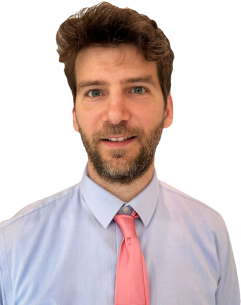MARKO ERCEGAssistant professor at the Departmant of Mathematics, Faculty of Science, University of Zagreb.

Research interest

PDEs and functional analysis: microlocal analysis (semiclassical measures, H-measures and variants), Friedrichs systems, operator theory.

Teaching

Applied mathematical analysis, Methods in mathematical physics, Mathematics (for biologists), Variational calculus and Convex analysis.

Friedrichs systems in a Hilbert space framework: solvability and multiplicity

We present a purely operator-theoretic description of abstract Friedrichs systems via the universal operator extension theory of dual pairs (Grubb, 1968). For a given Friedrichs system the existence of a boundary condition such that the problem is well-posed is shown, as well as a classification of all such boundary conditions. [Read more]

Velocity averaging for diffusive transport equations with discontinuous flux

We consider a diffusive transport equation with discontinuous flux and prove the velocity averaging result under non-degeneracy conditions. As a corollary, we show the existence of a solution for the Cauchy problem for nonlinear degenerate parabolic equation with discontinuous flux. [Read more]